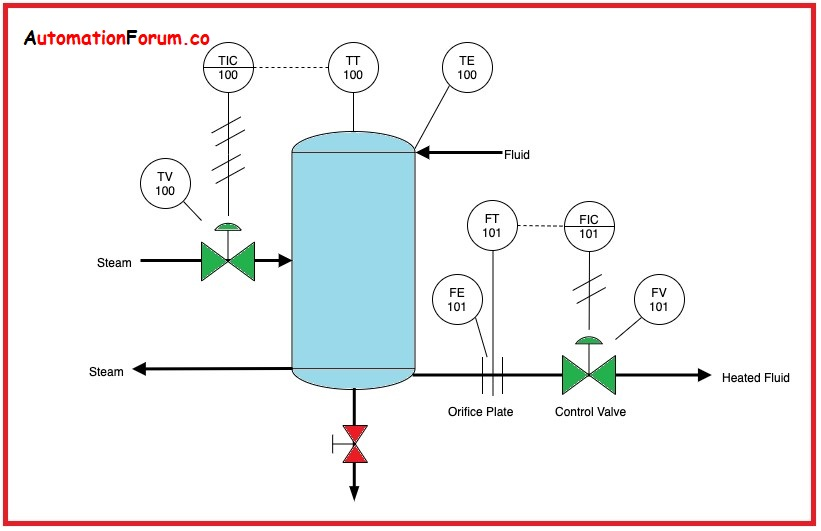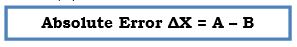# Instrumentation Basics Measurement Technology### What are the common measurement terminologies that are used in instrumentation?

• Measured Variable: It is the physical quantity or condition, which can be measured by the instrument. Measured variables such as temperature, pressure, rate of flow, level, speed, etc.
• Input Signal:  It is a signal applied to a device element or system. It is applied to the input terminals of an instrument. The signal may be 4-20mA or 3-15 psi.
• Output Signal: It is a signal delivered by a device element or system. The signal produced at the output connections of an instrument is referred output signal. The signal may be 4-20mA or 3-15 psi.
• Range:  It is the region between the limits within which a quantity is measured and transmitted. It is the region between maximum and minimum limit.  Ex, if the minimum value is 0 Degrees Celsius and the maximum value is 200 Degrees Celsius then the range value is max-min = 200-0 = 200 Degrees Celsius.
• Span: It is the algebraic difference between the upper range and lower range values.  Ex, the lower range is -20 Degrees Celsius and the upper range is 2000 Degrees Celsius.  Then the span is difference between upper range to lower range = 2000 – (-20) = 2020 Degrees Celsius.
• Suppressed zero: It  is used when a lower range value is greater than zero
• Elevated zero: It is used when the lower range value is less than zero.
• Error: It is the algebraic difference between the process variable and set point.
• Absolute Error: It is the algebraic difference between the indicated value (A) and the true value (B) of a quantity to be measured.

#• Relative Error: It is the ratio between the absolute error and the true value of the quantity to be measured.
• Resolution: It is the difference between any two adjacent values within a range. It is the smallest interval that can be identified as a measurement varies.
• Dead Band: It is the range at which an input is changing without observing output response or the case where no output exists.
• Correction Factor:  It is the algebraic difference between the true value and indicated value.
• True value: It is the actual magnitude of the input signal to the measuring system which can only be approached and never be evaluated.
• Sensitivity: It is defined as the ratio of change in output of the transducer corresponding to change in the measured value.
• Repeatability: It is the closeness of agreement among several independent measurements of the output for the same value of input under specified conditions.
• Reproducibility: The closeness of agreement among repeated measurements of output for the same value of input made under the same operating condition over a period that approaches in both directions.
• Ambient: It is the surrounding or environment about a particular point or object.
• Accuracy: The accuracy in an instrument is determined by the maximum amount by which the result of an instrument lies to the true value.
• Linearity: It is a closeness to which an output curve approaches a straight line.
• Hysteresis: It is the difference in the output for a given input. When the input of any instrument is slowly varied from zero to full scale and again back to zero, the output is varied.
• Gain: It is the ratio of change of the output to the change in the input applied. It is a special case of sensitivity, the gain is said to be unitless where the units for the input and output are identical.
• Uncertainty: It is the range of value within which the true value lies with a specified probability.  Uncertainty of +1% at 95 % confidence means the instrument will give the user a  range of +1% for 95 readings out of 100.
• Response: It is defined as the device output expressed as a function of time. It is the time taken by the device to respond to suitable information.
• Attenuation:  It is defined as a decrease in signal magnitude over some time.
• Calibration: It is the procedure of comparing and determining the performance and accuracy of an instrument called calibration.
• Closed-loop: It relates to a control loop where the process variable is used to calculate the controller output. In a closed-loop system, the control action is independent of the desired output.
• Hunting: Generally it is an undesirable oscillation near the required set point. Hunting generally arises when the demands exceed the performance of the system and the system capabilities are also increased. The controller output can be controlled due to the resolution of accuracy limitations.
• Ramp: It defines the delayed and accumulated response of the output to a sudden change in the input.
• Reliability: It is the probability that a device will perform within its specifications for the number of operations for a specific period.
• Set point: It is the variable that is given by the user to the instrument depending upon the process’s nature. It is represented in the units of the process variable and is used by the controller to determine the output of the process.
• Steady-state: It is used in closed-loop control where the process doesn’t oscillate or change and settles at some defined value.
• Time constant:  The time constant of a first-order system is defined as the time taken for the output to reach 65% of the total change when subjected to a step input change.
• Transient: It is a sudden change in a variable that is neither a controlled response nor long-lasting.
• Transducer: It is an instrument that converts information from one form into another. Basically from physical to electrical.
• Indicator: It is a device made to display an actual value of a process variable.  It may be digital or analogical.
• Recorder: It is a storage device that records and stores an actual value or process variable. Through this device the data can be accessed at any time.
• Controller:  It is a device that compares the process variable with the set point and acts accordingly to regulate a controlled variable in a specific manner.
• Auto manual station: It is a type of control station that allows switching between auto and manual modes.
• Control valve: It is a device that controls or regulates the flow of fluid through the line as per requirement. It is also known as the final control element.
• Amplifier: It is a device that enables an input signal to control a signal source independent of the signal and is thus capable of delivering an output that is related to and is greater than the input signal.
• Actuator: An actuator is the part of the final control device that causes a physical change in the final control device when signalled to do so.
• Field device: It is an instrument mounted on a process station with necessary mountings and connectors, these field devices includes sensors, transducers, and final control elements.

### What is instrumentation?

Instrumentation is the art and science that deals with the study of various field instruments that measure and controls the various process parameters like flow, level, temperature, and pressure.

### What are the types of signals used in instrumentation?

Pneumatic signals are signals produced by changing the air pressure in a signal pipe in proportion to the measured change in a process variable.

3-15 PSI

Electric signals are analogical signals produced by changing the electrical property in proportion to the change in a process variable. 4-20 ma,1-5 volt.

### What is a closed-loop?

A Closed control loop exists where a process variable is measured and compared with a set point and the corresponding action is taken to correct any deviation from the set point.

### What is the open-loop?

An open loop is a manual control loop that exists where the process variable is not compared with the set point.

### What is the P and I diagram in instrumentation?

1. This is a schematic drawing to show the process flow and its control.
2. P and I diagram is a very essential drawing for process measurement and control.
3. This is a diagram that shows the pipeline sketch along with the installed equipment, instrumentation, and control interlocks.
4. A standard set of symbols is used to represent all the components.
5. All the components of a P & I diagram are identified by numbering systems with its unique item number of every equipment and pipeline which can be identified easily.
6. The pipeline number contains its size, thickness, material, and fluid contents. Some suitable symbols can also identify the location of instruments and their type.

Scroll to Top